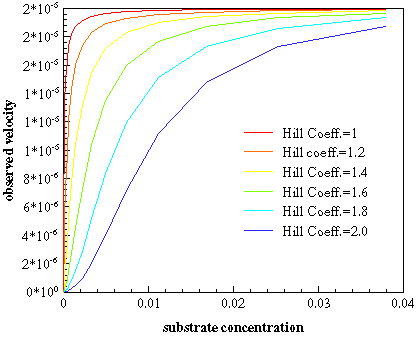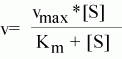The Effect of Varying the Hill Coefficient on Observed KineticsNormal Michaelis / Menten kinetics equation as modified with H=Hill coefficient H > 1 produces the simoid curve. Note: if H=1 it is the same as the eq. to the left!

The hill equation is the same as the basic michaelis-Menten equation with one modification. The [S] terms are raised to the power of H. In a perfectly cooperative enzyme H= the number of subunits.
Enzymes are rarley perfect, however and H can be any number from 1 to the number of subunits.
The subunits can exist in one of two slightly differnt conformations. a Hill coefficient = 1 means that the conformation of one subunit has NO effect on the conformation of the neighbors within the same molecule. A Hill coeeficient of greater than 1 means the opposite. the conformation in one subunit Does effect the neighbors.

The graph above demonstrates the effect of increasing amount of cooperativity within an enzyme as the hill coefficient varies from 1 to 2 within a dimeric protein.